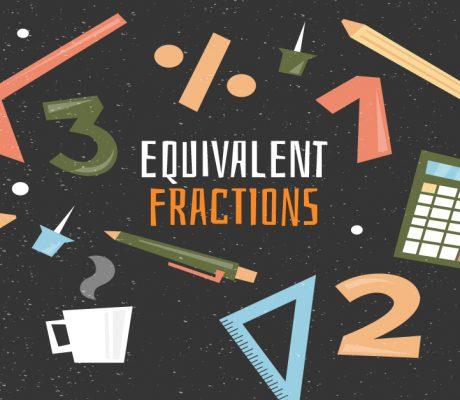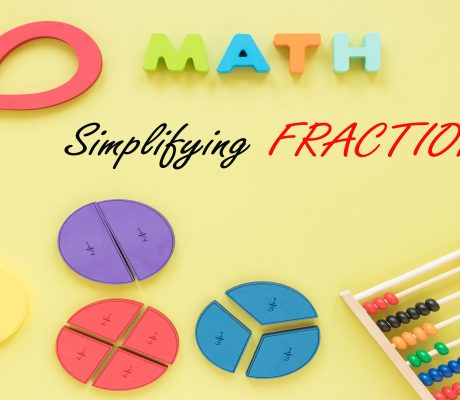### Equivalent Fractions

Equivalent fractions are fractions that represent the same size even if the numbers look different. Let’s take a look at the pizzas below. The two fractions have different numerators and denominators, but they represent the same size of pizza slices. when simplified, is actually . It is important to remember that these two fractions still […]### Learn ZOE Math Questions with Answers

In this blog, there are 21 math questions that Learn ZOE teachers prepared for you. The questions are based on different grade levels and once the answer is revealed, there will be a detailed explanation of how we got the correct answer. Feel free to answer the questions first before revealing the answers. Kindergarten Questions […]### Simplifying Fractions

What is a Fraction? A fraction represents a part of a whole. It describes equal parts of an object or a group like one-half, one-fourth, two-eighths, etc. For example, a slice of pizza is a fraction of the whole pizza. The top number (numerator) tells us how many parts we have. The bottom number (denominator) […]